A General Design Procedure for Bandpass Filters Derived from Low Pass Prototype Elements: Part I

K.V. Puglia
M/A-COM Inc.
Lowell, MA

Bandpass filters serve a variety of functions in communication, radar and instrumentation subsystems. Of the available techniques for the design of bandpass filters, those techniques based upon the low pass elements of a prototype filter have yielded successful results in a wide range of applications. The low pass prototype elements are the normalized values of the circuit components of a filter that have been synthesized for a unique passband response, and in some cases, a unique out-of-band response. The low pass prototype elements are available to the designer in a number of tabulated sources 1,2,3 and are generally given in a normalized format, that is, mathematically related to a parameter of the filter prototype.

This article presents a general design procedure for bandpass filters derived from low pass prototype filters, which have been synthesized for a unique filter parameter. A number of illustrated examples are offered to validate the design procedure.

LOW PASS PROTOTYPE FILTERS

Low pass prototype filters are lumped element networks that have been synthesized to provide a desired filter transfer function. The element values have been normalized with respect to one or more filter design parameters (cutoff frequency, for example) to offer the greatest flexibility, ease of use and tabulation. The elements of the low pass prototype filter are the capacitors and inductors of the ladder networks of the synthesized filter networks as shown in Figure 1. This diagram also depicts the two possible implementations of the low pass prototype filter topologies. In both cases, the network transfer function isT(s) = e2(s) / e1(s)

where

s = s + jw , the Laplace complex frequency variable

Clearly, the transfer function, T(s), is a polynomial of order n, where n is the number of elements of the low pass filter prototype.

The illustrated circuit topologies represent a filter prototype containing an odd number of circuit elements. To represent an even number of elements of the prototype filter, simply remove the last capacitor or inductor of the ladder network.

For purposes of illustration, an example representing a Chebyshev filter is offered. The power transfer function of the Chebyshev filter may be represented by

T(f') = 10log{1 + e cos2 [ncos-1 (f')]}

for f' > 1.0

T(f') = 10log{1 + e cosh2 [ncosh­-1 (f')]}

for f' > 1.0

and

ε = log-1(rdB / 10) – 1

where

rdB          = inband ripple factor in decibels

These equations represent the power transfer function of the Chebyshev low pass prototype filter with normalized filter cutoff frequency f' of 1.0 Hz. A graphical representation of the power transfer function of the Chebyshev low pass prototype filter is shown in Figure 2.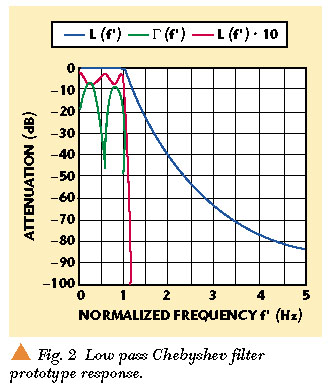The low pass prototype filter parameters for the low pass Chebyshev filter example are

n = 5

R'0 = 1.0 W

and

rdB = 0.5

A schematic representation of the prototype Chebyshev filter is shown in Figure 3. The prototype elements are from Matthaei, Young and Jones1 where the normalized cutoff frequency is given in the radian format w '1 = 1.0 = 2p f'1 .If this five-section prototype filter were constructed from available tables of elements and a circuit simulation performed, the transfer function would be exactly as represented in the schematic. To construct the filter at another frequency (1.0 GHz, for example) and circuit impedance level (R0 = 50 W ), the element values must be adjusted (de-normalized) in accordance withIn addition to the tabulated data of low pass prototype filter elements, the values may be computed via execution of the equations found in Matthaei, et al.1 , and repeated in Appendix A for convenience.

The schematic of the filter, which was derived from Chebyshev, the low pass prototype elements and the associated frequency response are shown in Figure 4. Note that the 0.5 dB in-band ripple results in a return loss of -10 dB as expected.A low pass filter may be converted to a bandpass filter by employing a suitable mapping function. A mapping function is simply a mathematical change of variables such that a transfer function may be shifted in frequency. The mapping function may be intuitively or mathematically derived. A known low pass to bandpass mapping function may be illustrated mathematically aswhere

 f0 = √f1 f2 D f = f2 – f1

and f0 , f1 and f2 represent the center, lower cutoff and higher cutoff frequencies of the corresponding bandpass filter, respectively. If the substitution of variables is made within the Chebyshev power transfer function, the power transfer function of the corresponding bandpass filter may be determined as shown in Figure 5.The schematic diagram of the bandpass filter, which was derived from the low pass prototype filter via the introduction of complementary elements and producing shunt and series resonators, is shown in Figure 6. This is a basic low pass to bandpass transformation, and unfortunately sometimes leads to component values, which are not readily available or have excessive loss. As described later, the mapping function need not be considered as part of the bandpass filter design procedure. It is presented here as a supplement to the filter theory.It bears repeating that the low pass prototype filter elements, that is, the g-values, are the result of network synthesis techniques to produce a desired characteristic of the prototype filter transfer function. These desired characteristics might include a flat amplitude response, maximum out-of-band rejection, linear phase response, Gaussian or other amplitude response, minimum time sidelobes and matched signal filters.

RF AND MICROWAVE RESONATORS

RF and microwave resonators are lumped element networks or distributed circuit structures that exhibit minimum or maximum real impedance at a single frequency or at multiple frequencies. The resonant frequency f0 is the frequency at which the input impedance or admittance is real. The resonant frequency may be further defined in terms of a series or shunt mode of resonance; the series mode is associated with small values of input resistance at the resonant frequency, while the shunt mode is associated with large values of resistance at the resonant frequency. Some typical lumped and distributed resonators are shown in Figure 7.Resonators may be characterized by their unloaded quality factor Qu , which is the ratio of the energy stored to the energy dissipated per cycle of the resonant frequency. Resonators are also characterized with respect to their reactance a or susceptance b slope parameters, which are defined, respectively, asThese are important resonator parameters because they influence Qu and the coupling factor between resonators in multiple resonator filters. Table 1 provides the reactance and susceptance slope parameters of some common lumped element and distributed resonators.

Qu may also be defined in terms of the reactance or susceptance slope parameter as

Q= α / Rse = ßRsh

where

 Rse = resonator series resistance Rsh = resonator shunt resistance

Together these resistances represent the resonator loss. The bandpass filter design examples will illustrate the utility of the slope parameters.

In many bandpass filter applications, particularly those applications where the filter is deployed at the front end of a receiver, it is important to know the Qu for the resonators in order to accurately estimate the insertion loss of the filter. The insertion loss of a single transmission resonator can be mathematically represented asAt f = f0 , this equation may further reduce to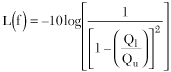Solving for Qu , yieldsTherefore, a measurement of the single resonator insertion loss at the resonant frequency L(f0 ) and the -3 dB bandwidth D f is sufficient to accurately determine Qu of any resonant structure. The loaded quality factor Ql may be determined from a measurement of the resonant frequency and the -3 dB bandwidth from the equation

where

Q1 = f0Df

D f = -3 dB bandwidth

This measurement technique may also be employed to compare the quality factors (Qu ) of different types of resonant structures or as a method of comparing various plating or manufacturing techniques for the filter if insertion loss is a critical parameter.

Consider an example. A PCS1900 transmit filter was required with minimum insertion loss and minimum size as critical design parameters. Rectangular, coaxial l /8 resonators were determined to offer the minimum volume in an eight-resonator filter consistent with the maximum insertion loss requirement of less than 1.0 dB at the center frequency of 1960 MHz. A single resonator was constructed of the type anticipated to be used within the filter. The single resonator is shown in Figure 8.A single resonator structure was fabricated and plated with silver in order to obtain the maximum Qu . The resonant structure was tuned to the desired center frequency and the insertion loss L(f0 ) and -3 dB bandwidth were measured. The measurement data is shown in Figure 9 whereL(f0 = 1.950 GHz) = 0.533 dB

D f = 14.50 MHz

Qu 2250.

In executing the measurement, three notes of caution are required in the interest of accuracy: The coupling probes to the cavity should be equal and minimized to avoid load and source resistance across the resonator; the source and load SWRs should be kept low; and the input SWR at f0 should be minimized to avoid mismatch loss.

The equivalent circuit of the single resonator is shown in Figure 10. Note that the circuit element, which represents the resonator loss Rsh , has been included. The value of Rsh may be determined with the aid of the susceptance slope parameter b fromQu = b Rsh

where

ß =

Y02 [(cot(θ0) + θ0csc2(θ0)] = 0.01836

for θp4 and Y170 Mhos

or

Rsh = 122.5 kW

This measurement technique for estimating the value of Qu is completely general and applies to lumped element and distributed resonators.

RESONATOR COUPLING

Resonator coupling represents one of the most significant factors affecting filter performance. There are several methods to couple resonators. For ease of manufacturing and tuning, a common resonator type and coupling method is generally preferable. Matthaei1 proposes what have been termed J (admittance) and K (impedance) inverters both to permit a common type of resonator and to serve as coupling elements for the resonators.

The J inverters may be represented as the admittance of the element or the value of the characteristic admittance of a quarter-wavelength line in the equivalent circuit that couples the resonators. Similarly, the K inverters may be represented as the impedance of the element or the value of the characteristic impedance of a quarter-wavelength line that couples the resonators. This permits the general expression of the coupling between resonators to be mathematically written as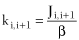and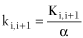for shunt-type and series-type resonators, respectively, where the coupling between the ith and the ith+1 resonators is represented by ki,i+1 .

A similar approach to the general design of bandpass filters employing common types of resonators proposes specific coupling elements in the case of lumped resonator bandpass filters or specific proximity methods of coupling in the case of distributed resonator bandpass filters. To illustrate, consider the coupled p -type of L-C resonators and the coupled l /8 transmission line distributed resonators shown in Figure 11.In the case of the coupled l -type L-C resonators, a series capacitor is inserted between the resonators to perform the coupling function, in which case the coupling coefficient is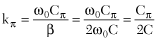In the case of the coupled l /8 transmission line resonators, the equivalent circuit shown in Figure 12 is useful in determining the coupling coefficient. The coupling coefficient may be determined from the capacitive matrix parameters associated with the coupled lines, that is, the capacitance per unit length to ground Cg and the mutual capacitance per unit length between the conductors Cm , where V is the velocity in the dielectric medium. The coupling coefficient may be written asFor the special case where θ0 = p /4, the coupling may be written asAnother very popular type of resonator, which is frequently used in microwave bandpass filters, is the quarter-wavelength resonator. Figure 13 illustrates the coupling of symmetrical l /4 resonators and the equivalent circuit. Note that l /4 resonators must be grounded at opposite ends to prevent the null coupling condition caused by cancellation of the electric and magnetic field modes.The coupling coefficient for this configuration may be written asFor a given coupled line geometry, the l /4 lines offer closer coupling than the comb-line configuration.

The input, output and adjacent resonator coupling in a multi-element bandpass filter are the parameters that determine the amplitude, phase and SWR over the passband of the filter. This statement understates the importance of resonator coupling to the bandpass filter parameters.

Recall that the elements of the low pass prototype filter, from which the bandpass filter is derived, determine completely the characteristics of the resulting filter. This fact will become evident when the coupling between resonators is disclosed to be a function only of the fractional bandwidth and the low pass filter prototype elements. Fortunately, a measurement technique is available to verify the coupling values of symmetrical resonators, and may also be utilized in multi-resonator filters. That measurement technique will now be explored.

The amplitude response of any pair of symmetrical resonators may be represented by1In the equation, k is the coupling coefficient between the symmetrical resonators, Qu is the unloaded quality factor of each resonator and Qe is the external quality factor. The external quality factor is defined to differentiate the source and load coupling and loss from the losses associated with the individual resonators (Qu ).

If the overcoupled condition is satisfied such thatit is possible to determine the resonator coupling coefficient from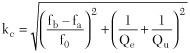where f0 , fa and fb are subsequently defined.

The utility of the equations will be demonstrated by two examples. Consider the symmetrical, lumped element resonators in the schematic shown in Figure 14 where two, p -type, L-C resonators are coupled by the capacitor Cp , and the external source and load are coupled to the respective resonators by capacitor Ce . Note also the Qu of each resonator isThe following variables have been assigned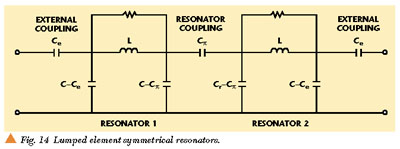With the assigned variables, the amplitude response is shown in Figure 15. The two peaks in the amplitude response correspond to the frequencies fa and fb . Using the assigned variables, the calculated coupling coefficient is kc = 0.05033.A second illustrative example using coupled l /8 resonators is shown in Figure 16. An equivalent circuit representation of the coupled lines, external coupling and resonator loss is required in order to quantify the coupling factor.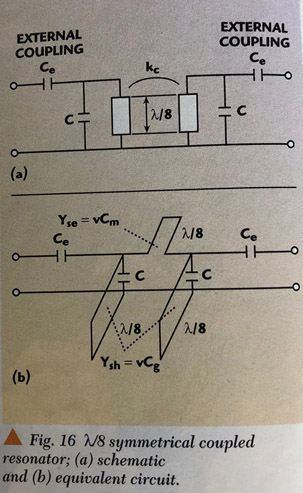The variables have been assigned as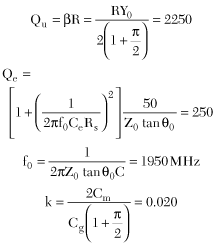With the assigned variables, the amplitude response is shown in Figure 17. The two peaks in the amplitude response correspond to the frequencies fa and fb . Using the assigned variables, the calculated coupling coefficient is kc = 0.01999. Part II explores how to use the preceding principles and data to design bandpass filters using a variety of lumped and distributed element resonators.ACKNOWLEDGMENT

The principal reference for the content of this article is the work of Matthaei, Young and Jones.1 Many of the concepts within this reference have been investigated and interpreted in order to provide a greater intuitive understanding of the bandpass filter design process. This text is strongly recommended for those having little familiarity with this work.

References

1. G.L. Matthaei, L. Young and E.M.T. Jones, Microwave Filters, Impedance-matching Networks and Coupling Structures, McGraw-Hill, New York, 1964.

2. E.G. Cristal, "Coupled Circular Cylindrical Rods Between Parallel Ground Planes," IEEE Transactions on Microwave Theory and Techniques, Vol. MTT-12, July 1964, pp. 428-439.

3. W.J. Getsinger, "Coupled Rectangular Bars Between Parallel Plates," IRE Transactions on Microwave Theory and Techniques, Vol. MTT-10, January 1962, pp. 65?72.

4. A. Zverev, Handbook of Filter Synthesis, John Wiley and Sons, New York, 1967.

5. G.L. Matthaei, "Interdigital Band Pass Filters," IEEE Transactions on Microwave Theory and Techniques, Vol. MTT-10, No. 6, November 1962.

6. G.L. Matthaei, "Comb-line Bandpass Filters of Narrow or Moderate Bandwidth," Microwave Journal, Vol. 6, No. 8, August 1963.

7. S.B. Cohn, "Parallel-coupled Transmission-line Resonator Filters," IEEE Transactions on Microwave Theory and Techniques, Vol. MTT-6, No. 2, April 1958.

8. R.M. Kurzrok, "Design of Comb-line Bandpass Filters," IEEE Transactions on Microwave Theory and Techniques, Vol. MTT-14, July 1966, pp. 351?353.

9. M. Dishal, "A Simple Design Procedure for Small Percentage Round Rod Interdigital Filters," IEEE Transactions on Microwave Theory and Techniques, Vol. MTT-13, September 1965, pp. 696?698.

10. S.B. Cohn, "Dissipation Loss in Multiple-coupled Resonator Filters," Proceedings of the IRE, Vol. 47, No. 8, August 1959, pp. 1342?1348.

Kenneth V. Puglia holds the title of Distinguished Fellow of Technology at the M/A-COM division of Tyco Electronics. He received the degrees of BSEE (1965) and MSEE (1971) from the University of Massachusetts and Northeastern University, respectively. He has worked in the field of microwave and millimeter-wave technology for 35 years, and has authored or co-authored over 30 technical papers in the field of microwave and millimeter-wave subsystems. Since joining M/A-COM in 1971, he has designed several microwave components and subsystems for a variety of signal generation and processing applications in the field of radar and communications systems. As part of a European assignment, he developed a high resolution radar sensor for a number of industrial and commercial applications. This sensor features the ability to determine object range, bearing and normal velocity in a multi-object, multi-sensor environment using very low transmit power. Puglia has been a member of the IEEE, Professional Group on Microwave Theory and Techniques since 1965.